• 3
•
• 1
•
• 1
•
•
•
5
Shares

# ELECTRIC CURRENT : THE BASIC CONCEPT

## INTRODUCTION

Electric current is one of the most basic concepts that exists in electrical physics. It is the key point within the science of electricity because every electrical equipments are running due to electric current. Without electricity whole world can go in darkness.

Everything, which you can see around your surroundings such as telephone, television, mobile phone, generator, fans, machine etc. All these are working due to electric current or electricity.

So every electrical machines are running due electric current. But have you ever seen electric current?. Offcourse not but may be you have seen some electrical sparks on the transformer due to heavy load.

But is this a electric current? Absolutely not, because sparking is not electric current it is only effect of electric current when wires get too close to each other. Sparking is just the form of energy in the form of light and sound. But it is not electric current.

So everyone ask me that what is electric current in really? Now in this section we are going to talk about electric current in detail, so stay tuned with us till end.

## ELECTRIC CURRENT : DEFINITION

An electric current is nothing but the flow of electric charge in a circuit. If we talk more formally, then we can say that the electric current is the rate of flow of charge to a given point in an electric circuit. This charge can be negatively charged electrons and positively charged protons, positive ions or holes.

The magnitude of electric current in any circuit is measured in coulomb per second. This coulomb per second is called the unit of electric current called Ampere or amp. It is denoted as capital A. It is widely used unit in electrical physics with many multipliers such as miliAmpere (mA) {0.001A}, microAmpere (μA) {0.000001A} and so on.

The electric current which flow through the circuit is normally denoted as I. The mathematical expression of electric current is given as –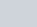If the small charge (dq) is flowing through a conductor in a small time (dt), then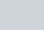Here,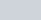is the first derivative of q with respect to t. It means electric current is the first derivative of q with respect to the time passing through any cross section of conductor in a particular direction. We can also express the electric current in the terms of electronic charge and number of charge carriers i.e electrons.

Let,
n = numbers of charge carriers
e = electronic charge
t = time
Then, electric current is given as-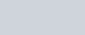• If q is positive, then the direction of electric current is forward
• But if q is negative, then the direction of electric current is backward.

## HOW ELECTRIC CURRENT GENERATED

Among all the solids, metals are good conductor of electricity. They can easily conduct electric current through them. But the question is how?

The electric conduction in the metals can be explained as follows :

We know that every substance is made up of atoms. In the nucleus of every atoms protons and neutrons co-exist and electrons keep revolving around this nucleus.

The electron which is nearest to the nucleus experiences the strong attractive force due to positive nuclear charge and the results electrons are bounded to it.

But the electrons which is far from the nucleus experiences a feeble force. So it is loosely bounded to the atom. And it can leave the atom any time when it gains little more energy from external means. Hence, the outer electrons can be easily removed from the nucleus just by rubbing or heating the substance.

When the outer electrons leave the atoms then it start moving randomly in the vacant space of the conductor. These electrons are called free electrons. Because it is free to move in the entire region of metallic conductor.

Electrons are negatively charged particles so when these free electrons moves in a particular direction then the electric current generated. Then we say material is electrically conductive.

Metallic conductors have a very large numbers of free electrons so it is regarded as good conductor of electricity.

The substance which have higher numbers of free electrons are good conductor of electricity but the substance which have lower numbers of electricity are comparatively bad conductor of electricity.

Gold and silver are best conductor of electricity but it is not uesd in wiring because it is very costly metals. So we use mostly copper and aluminium wire for electrical transmission.

In case of solid conductors (like copper, iron, gold and silver) atoms are tightly bound to the nucleus but there is a huge amount of free electrons are present in them. The motion of these free electrons are responsible for the generation of electric current, when electric field is applied.

Instead of solid conductors, some liquid and gases can also conduct electricity. But there is important difference between the electric conduction in metals and that in liquid and gases.

We know that in metals electric conduction is occurred by the motion of negatively charged particles i.e free electrons only. But in liquid and gases it is occurred by the motion of both positive and negative charge carriers i.e ions.

In case of a liquid conductor like electrolytic solutions, there is a positive and negative charges ions which can move when external electric field is applied.

There are many others materials which have electrons but they are bound to the nucleus and they can’t be accelerated, even if electric field is applied. Due to no acceleration there will be no electric current. Such materials are called insulators. E.g. wood, plastic, rubber etc.

## CURRENT DENSITY

Current density at a point in any conductor is the ratio of electric current to the cross sectional area of conductor at that point. If I is the electric current which is uniformly distributed all over the cross sectional area A of the conductor. Then in this case current density is given as-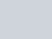It is the characteristics property of a point in the conductor. It is a vector quantity and it’s direction at a point is in the direction of motion of positive charge at that point.

### POINTS TO BE REMEMBERED

• When there is no electric field applied on a solid conductor then all the free electrons inside the conductor moves like the molecules of the gas due to their thermal velocities. This motion is called random motion. There is no prefered direction for the velocities of the free electrons.  The average thermal random velocity is zero. Due to this there is no net flow of electric charges in a particular direction inside the conductor and hence no electric current generated in it.
• When we applied a electric field on the solid conductor of a shape of right circular cylinder of uniform cross section area by attaching positively and negatively charged circular disk of a dielectric of the same radius as that of circular cylinder. Then at the both ends, a electric field is setup inside the conductor from the positive charged disk to the negative charged disk.Electric field applied to the cylindrical conductor. Image source : electrical classroom
• Due to this electric field, the free electrons inside the conductor accelerated towards the positive disk in order to neutralize the charge of the disk. This motion of the electrons remains continue till the effect of the electric field inside the conductor exists. In this situation, a electric current is generated for a short period of time inside the conductor this current is called transient current.
• If a electrolytic solutions have positive and negatively charged ions then it can easily conduct electricity.

For more educational content, subscribe to our newsletter.